# Riesz decomposition property

(diff) ← Older revision | Latest revision (diff) | Newer revision → (diff)

Let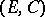be a partially ordered vector space, [a5], i.e.is a real vector space with a convex conedefining the partial order by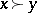if and only if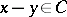. For, the corresponding interval is.

The (partially) ordered vector spacehas the Riesz decomposition property iffor all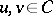, or, equivalently, if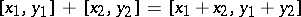for all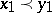,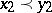.

A Riesz space (or vector lattice) automatically has the Riesz decomposition property.

Terminology on this concept varies a bit: in [a2] the property is referred to as the dominated decomposition property, while in [a3] it is called the decomposition property of F. Riesz.

The Riesz decomposition property and the Riesz decomposition theorem are quite different (although there is a link) and, in fact, the property does turn up as an axiom used in axiomatic potential theory (see also Potential theory, abstract), see [a1], where it is called the axiom of natural decomposition.

There is a natural non-commutative generalization to the setting of-algebras, as follows, [a4]. Let,,be elements of a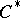-algebra. If, then there aresuch that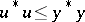,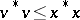and.

How to Cite This Entry:
Riesz decomposition property. Encyclopedia of Mathematics. URL: http://encyclopediaofmath.org/index.php?title=Riesz_decomposition_property&oldid=18014
This article was adapted from an original article by M. Hazewinkel (originator), which appeared in Encyclopedia of Mathematics - ISBN 1402006098. See original article